# NCERT Solutions for Class 10 Maths Chapter 5 Arithmetic Progressions

Chapter 5 Arithmetic Progression:

5.1 Introduction Sub-topics of Class 10

In this chapter, we’ll look at patterns that we see in our daily lives where the following terms are obtained by adding a set number to the previous terms. We’ll also learn how to calculate their nth terms and the sum of n consecutive terms, as well as how to apply this information to real-world challenges.

5.2 Arithmetic Progressions

The topic introduces Arithmetic Progressions, as well as its definition and related terminology.

Arithmetic Progression

The article discusses Arithmetic Progressions, including its definition and related words, as well as some excellent examples. Finite Arithmetic Progressions and Infinite Arithmetic Progressions will also be covered. AP takes the following forms: a, a + d, a + 2d, a + 3d,…

5.3 An AP’s nth Term

The numerous ways for determining the nth phrase of an AP are discussed in this topic. Different forms of problem-solving approaches and finding the nth term of an AP are used to clarify the ideas. The examples in this chapter will assist you in completing the exercise problems.

5.4 Sum of an AP’s First n Terms

The subjects cover various methods for calculating the sum of an AP’s first n terms.

5.5 Conclusion

It provides an overview of the entire chapter as well as the most essential subjects covered. You can cover the entire chapter in a few points by going through the summary section, which will aid in memorizing the key principles.

NCERT Solutions for Class 10 Maths Chapter 5 Arithmetic Progressions | Free PDF Download

This NCERT Solutions for Class 10 Maths is an excellent study resource.

This NCERT Solutions for Class 10 Maths is an excellent study resource that will assist you in solving a variety of issues. Solving these NCERT Solutions will assist you in fully comprehending the topic and laying a stronger foundation for future study.

Students will explore patterns in subsequent terms that are obtained by adding a fixed number to the preceding terms in this chapter. They also learn how to calculate the sum of n consecutive terms and the nth term. When students tackle real-life issues, they will successfully understand arithmetic progression.

This chapter discusses the Arithmetic Progression Derivation of the nth term and the sum of the first n terms of an A.P., as well as their application to everyday problems. This is an important chapter from the book.

From the standpoint of the Class 10 examination, this is one of the most essential chapters. Arithmetic progression is a very basic and crucial topic to understand because arithmetic progression will be asked in practically all competitive exams.

What are the types of questions in NCERT Solutions for Class 10 Maths Chapter 5?

Multiple choice questions, descriptive type questions, long answer type questions, short answer type questions, fill in the gaps, and daily life examples are all included in NCERT Solutions for Class 10 Maths Chapter 5. Children’s will be able to improve their problem-solving and time management skills by the end of this chapter. This helps them get good grades in their CBSE board exams 2023.

Is it required to learn all of the concepts covered in Chapter 5 AP of the NCERT Solutions for Class 10 Maths?

Yes, all of the concepts in NCERT Solutions for Class 10 For the advantage of students studying for the CBSE Class 10 Maths test, NCERT Solutions for Class 10 Maths Chapter 5 Arithmetic Progression are offered here. It is critical for students to become familiar with these NCERT solutions in order to achieve a good grade in the Class 10 examination. Our specialists answer these NCERT difficulties. These answers will assist you in comprehending and mastering various types of arithmetic progressions questions. NCERT Solutions assist you in achieving proficiency in the solving of all types of queries.

This chapter is part of unit 3 algebra, which has a total of 20 points in the exam. From arithmetic progressions, students should expect an average of three questions. This topic is particularly essential from the standpoint of competitive tests, in addition to Class 10 examinations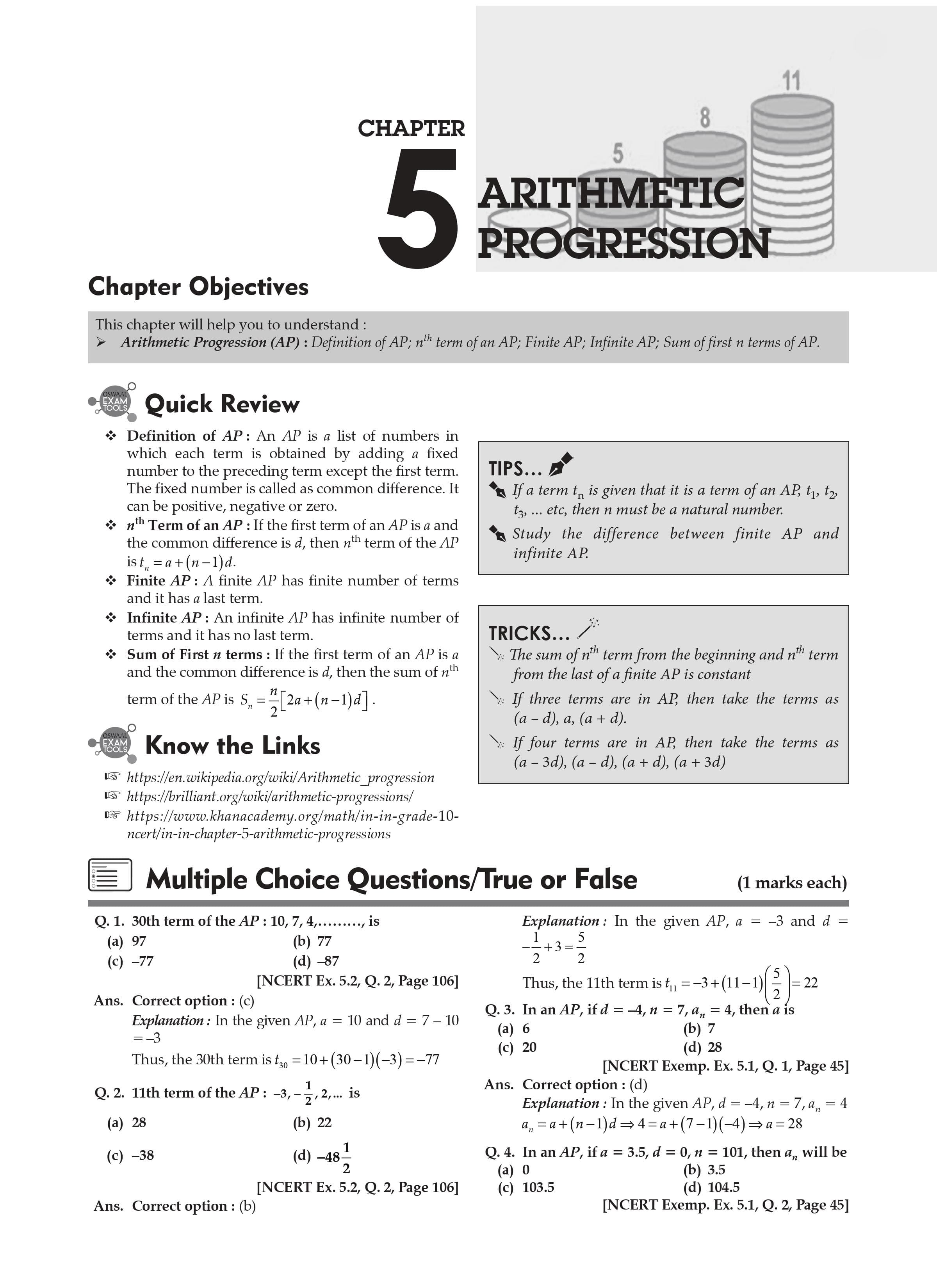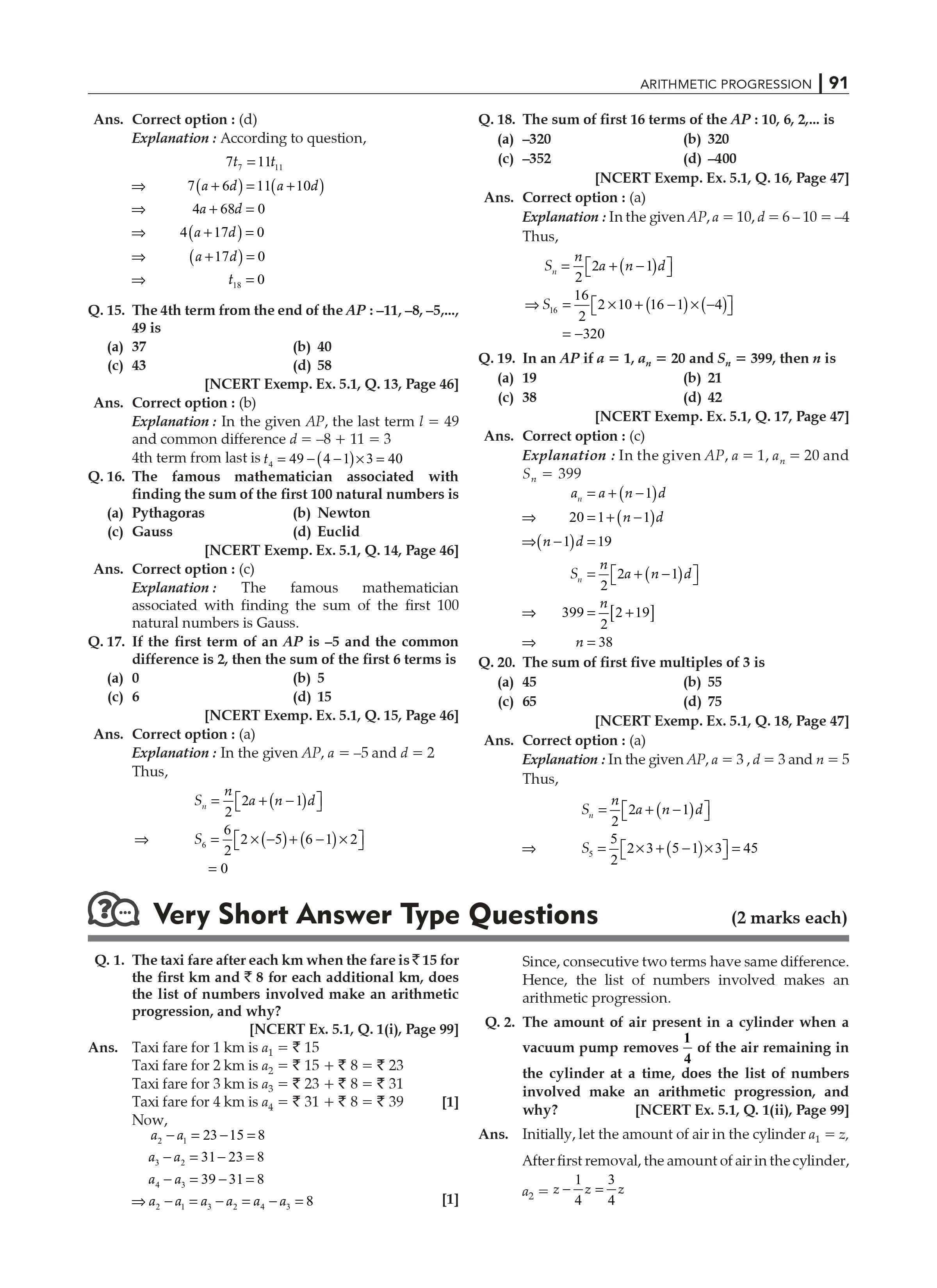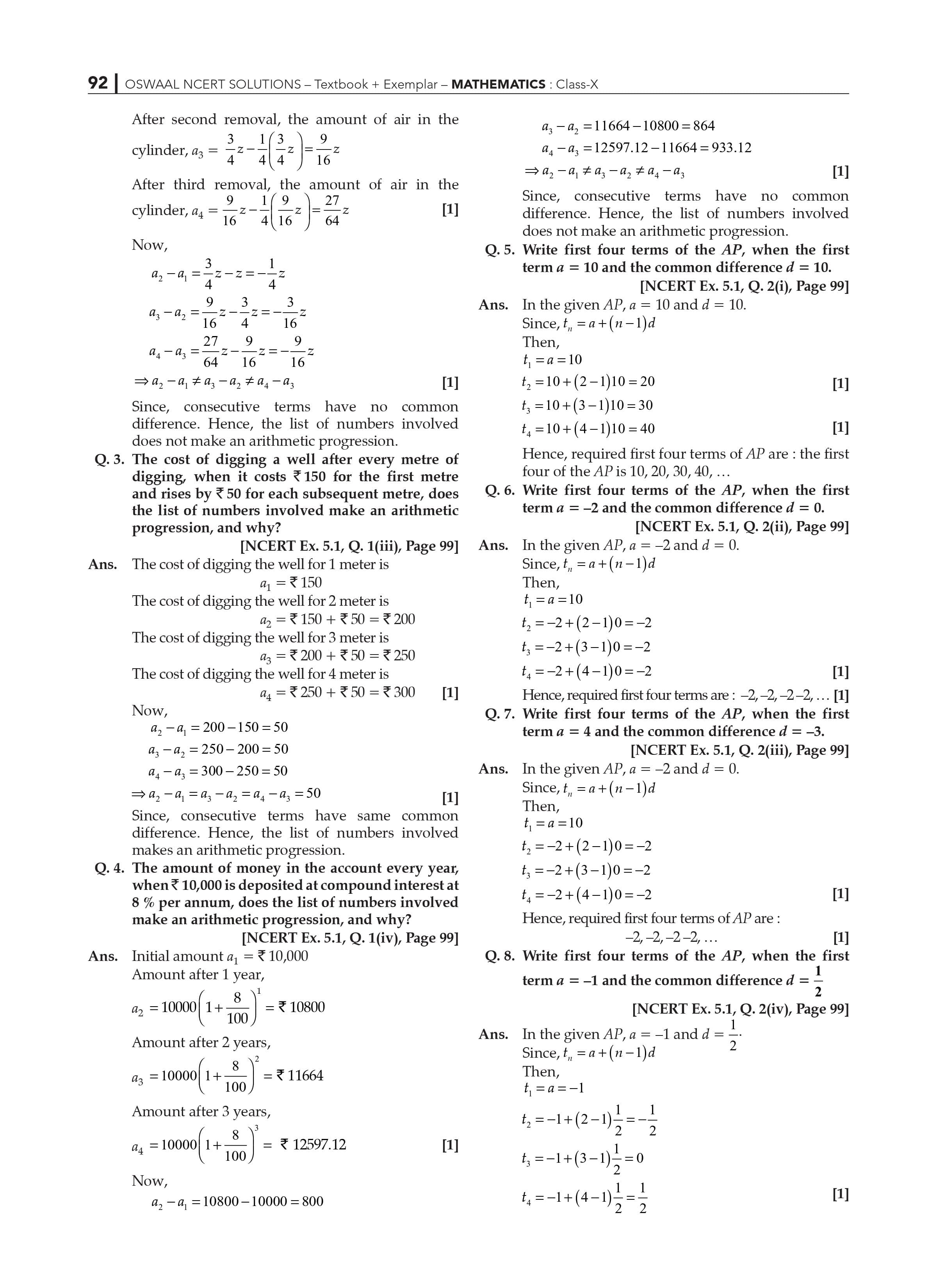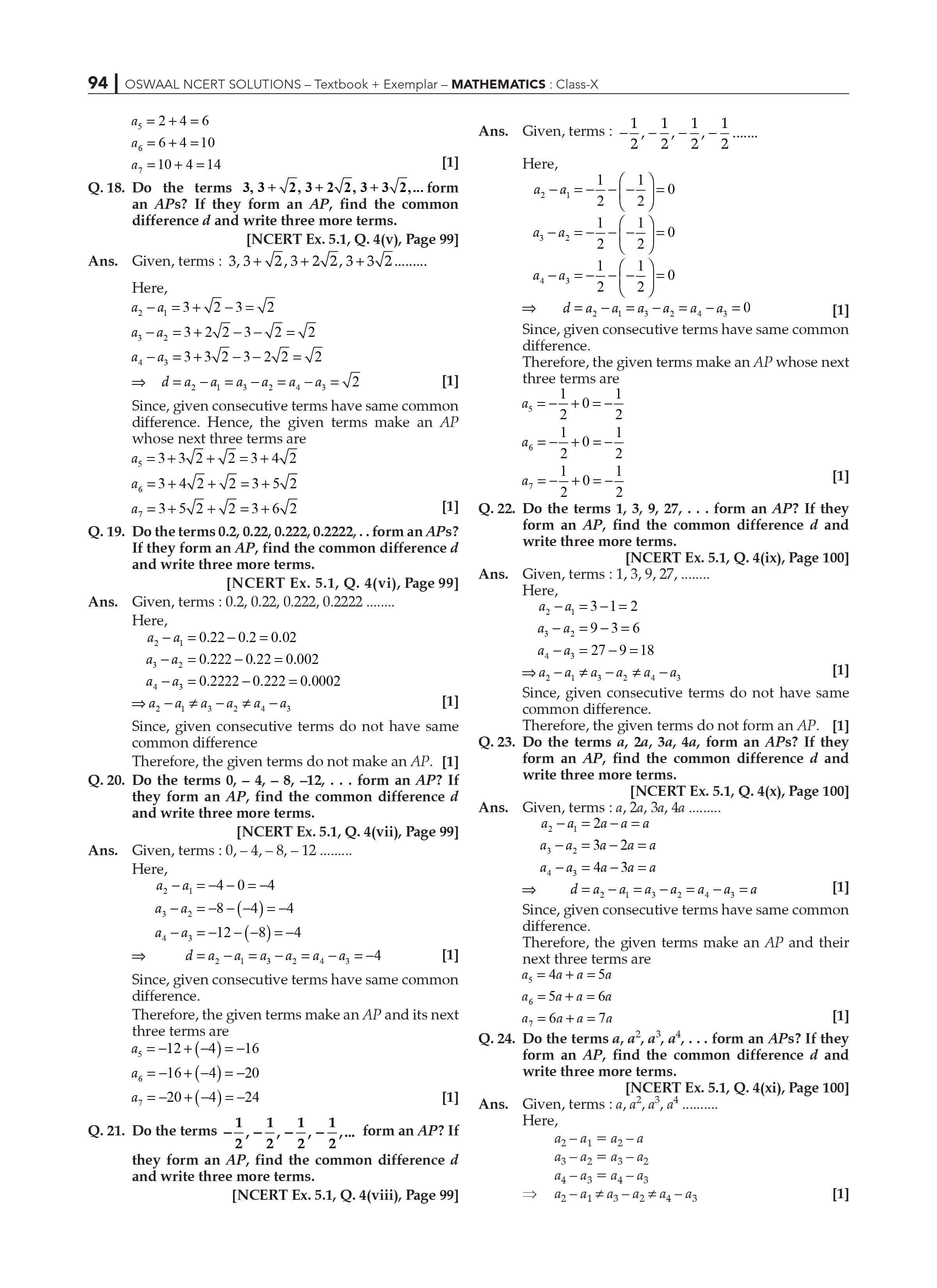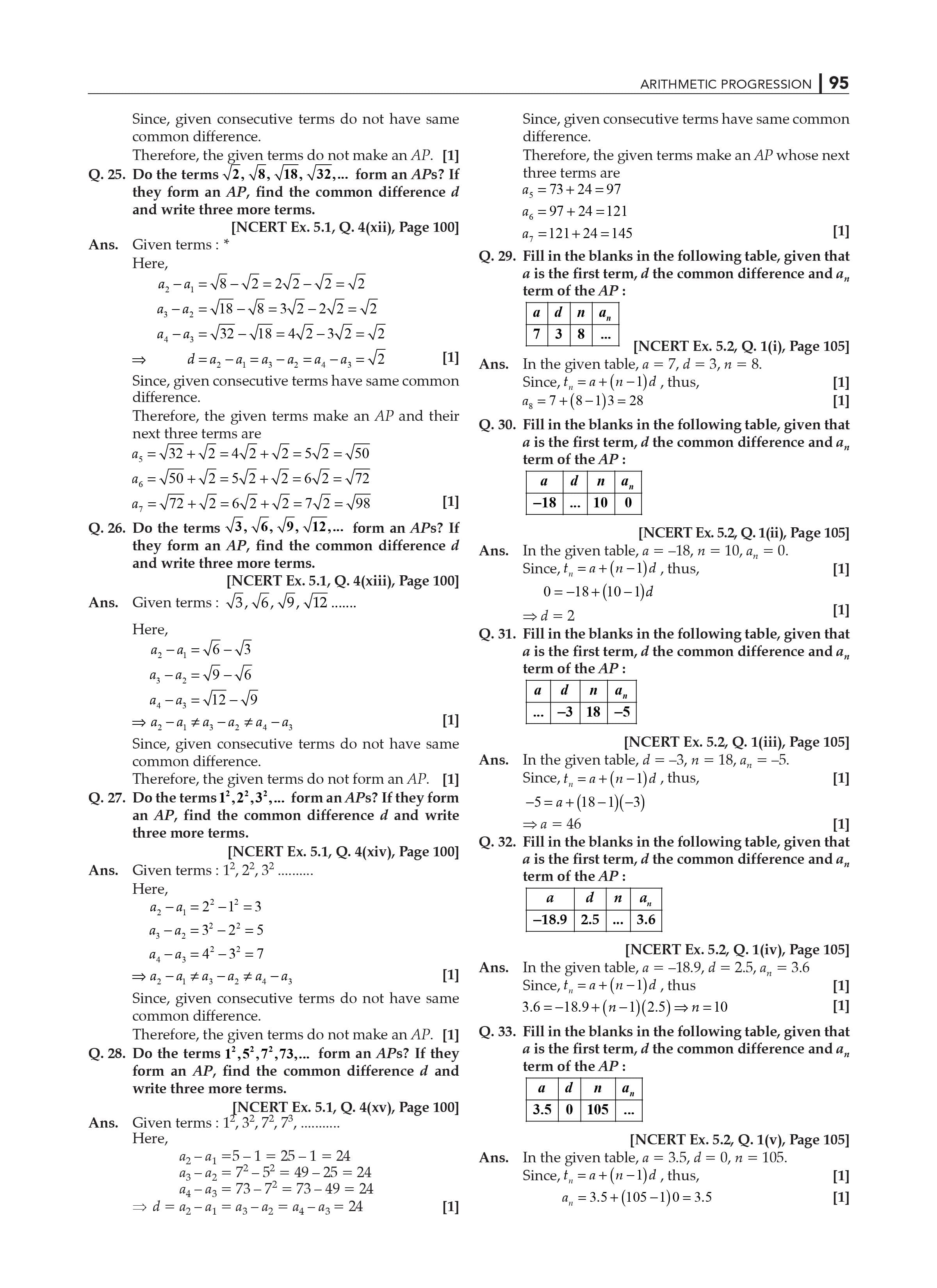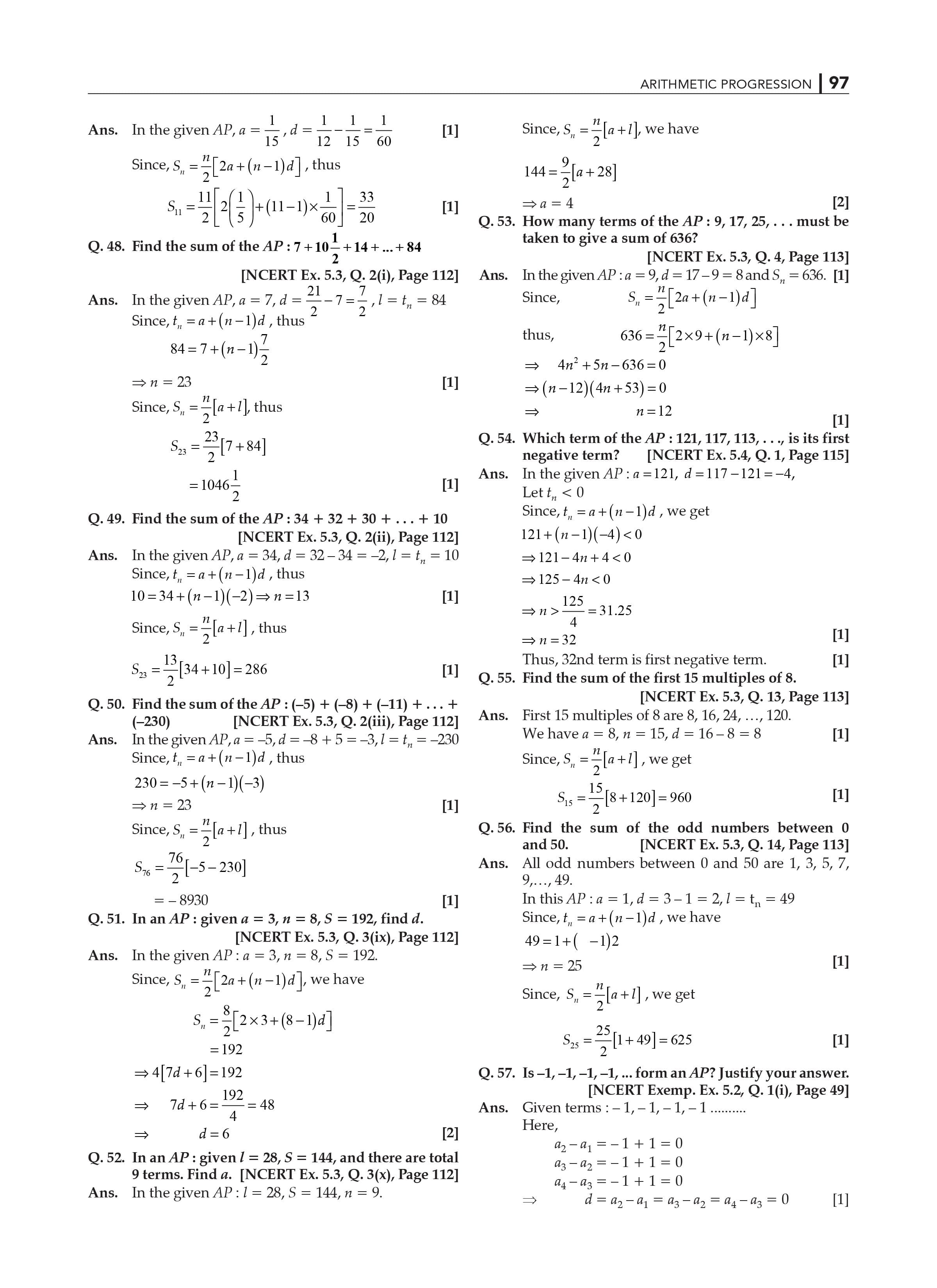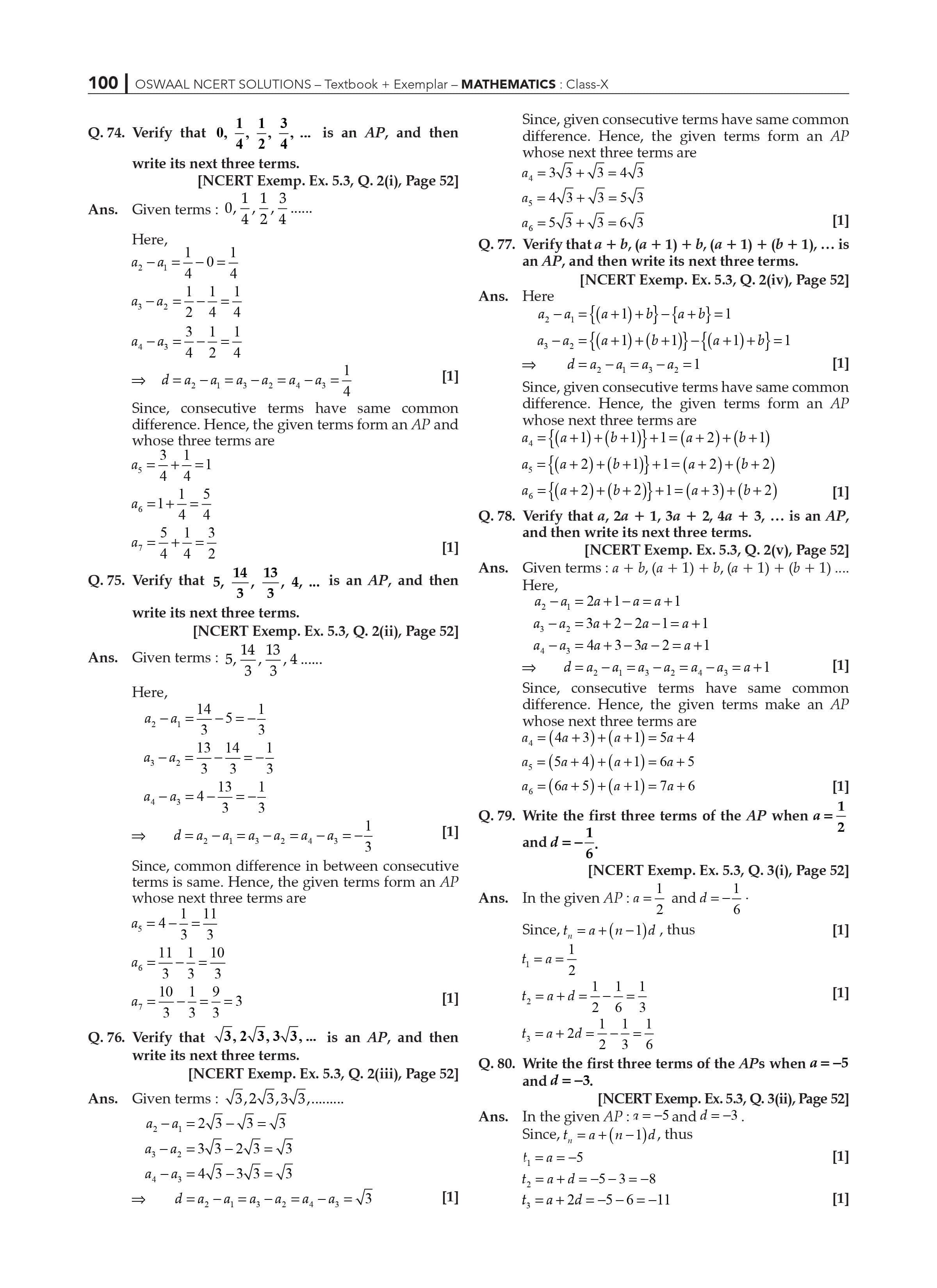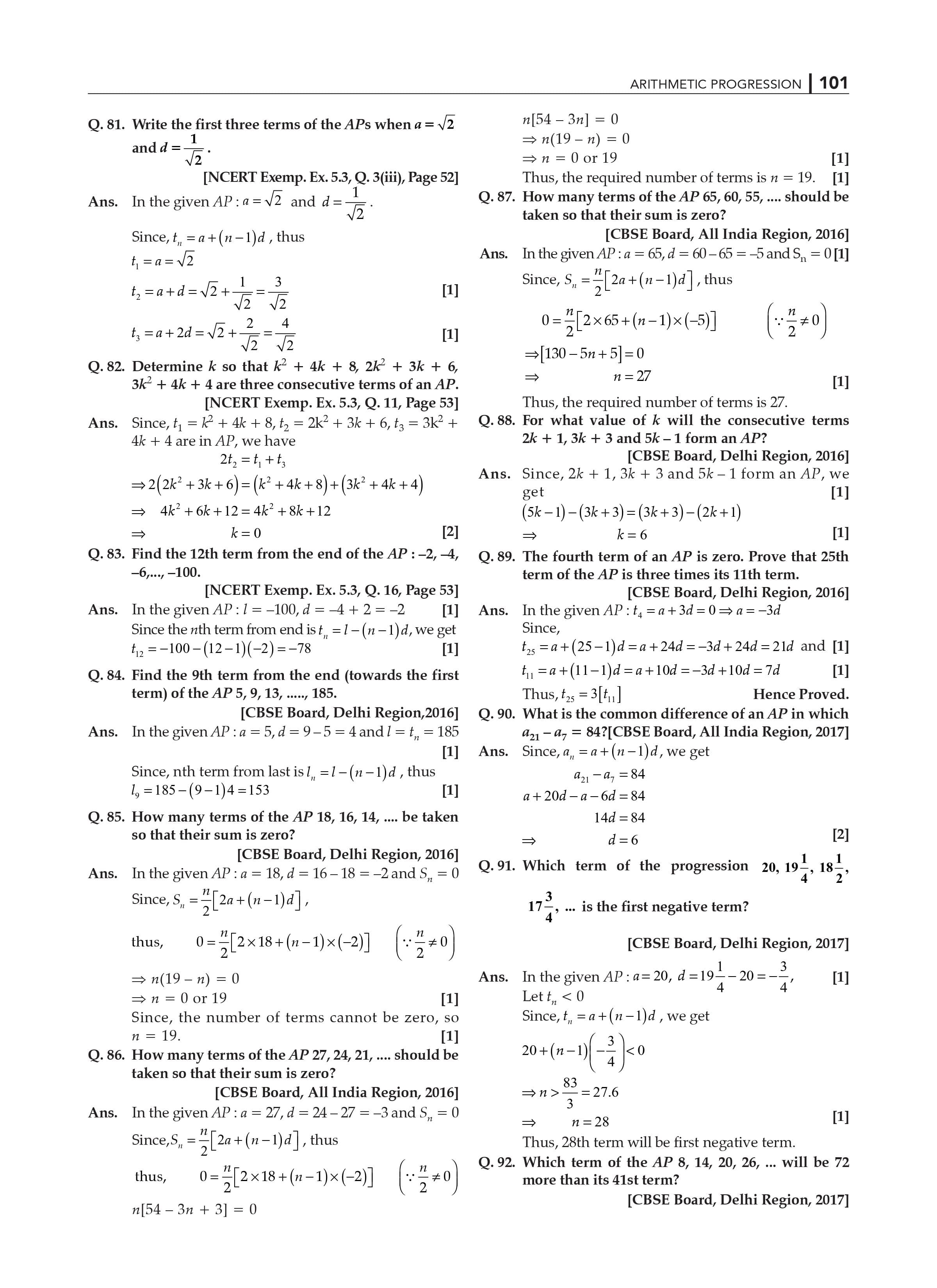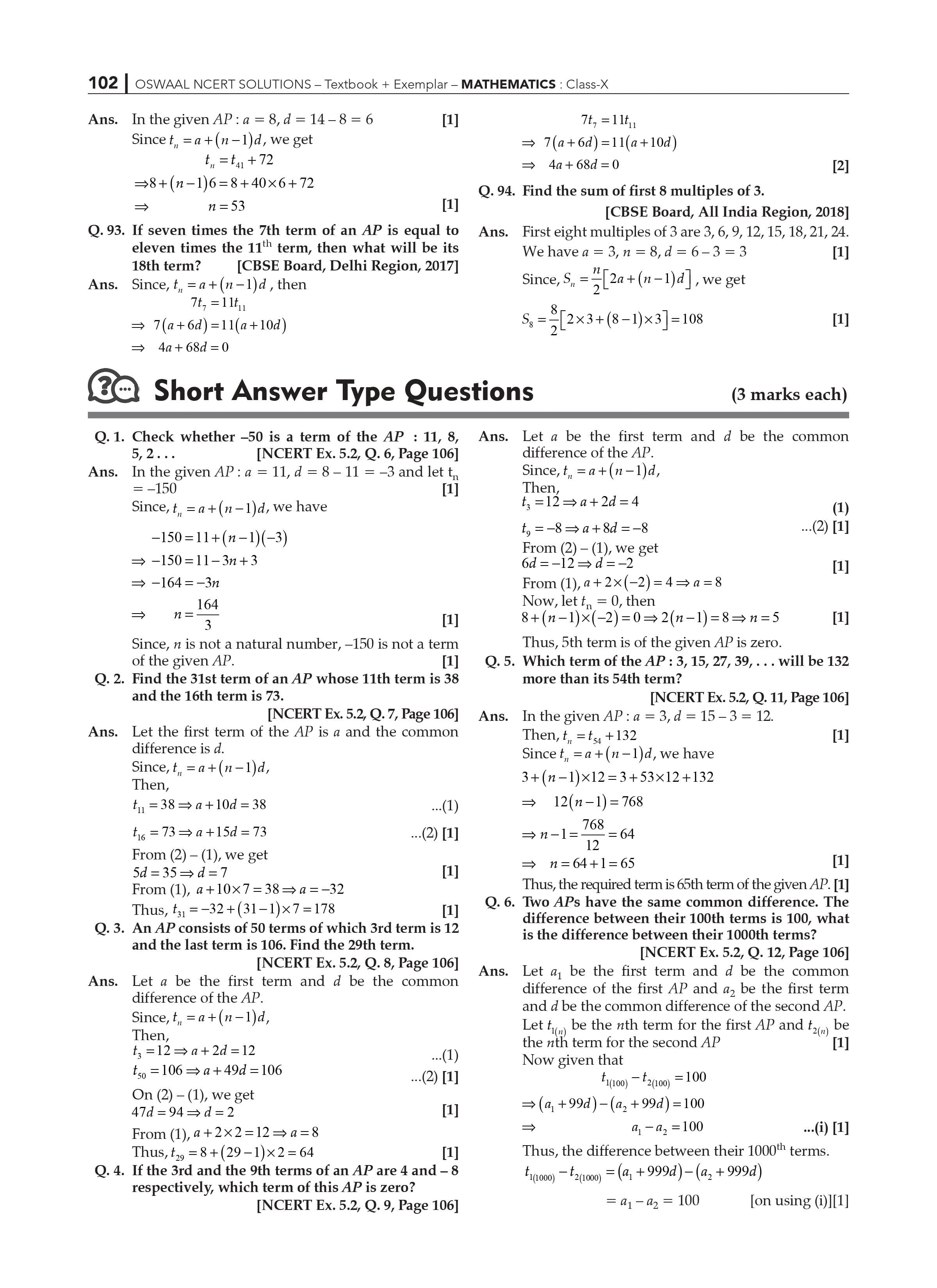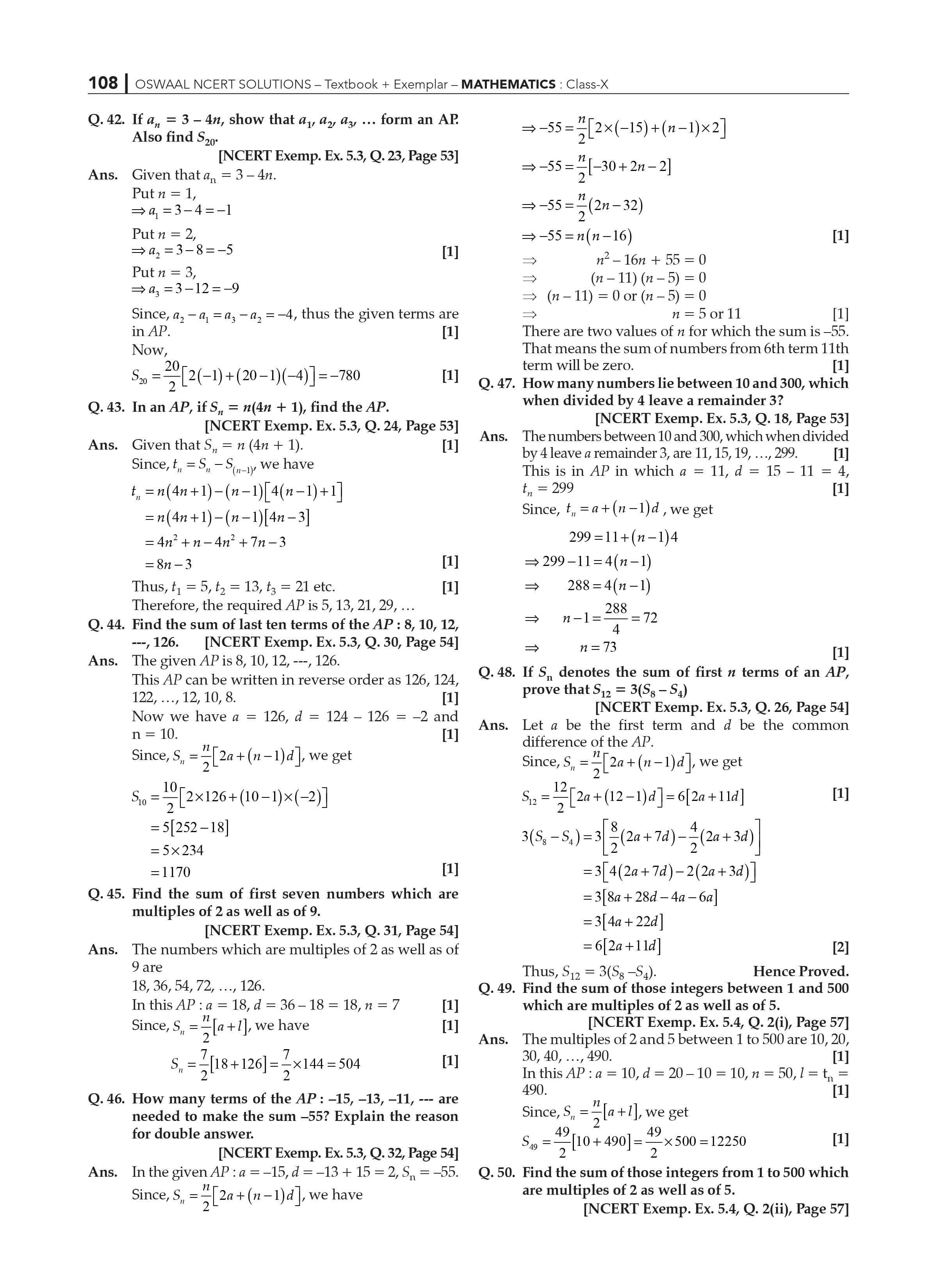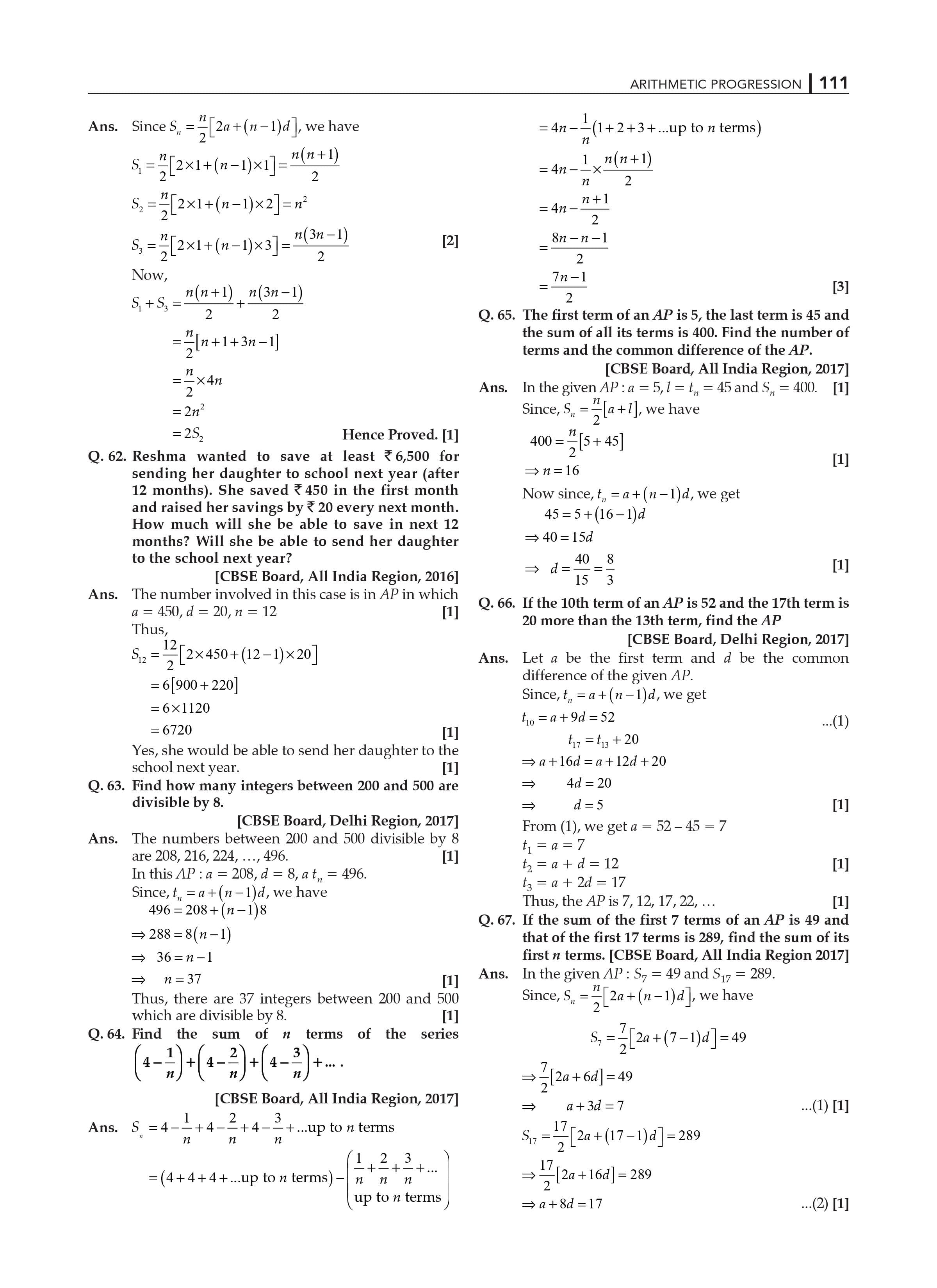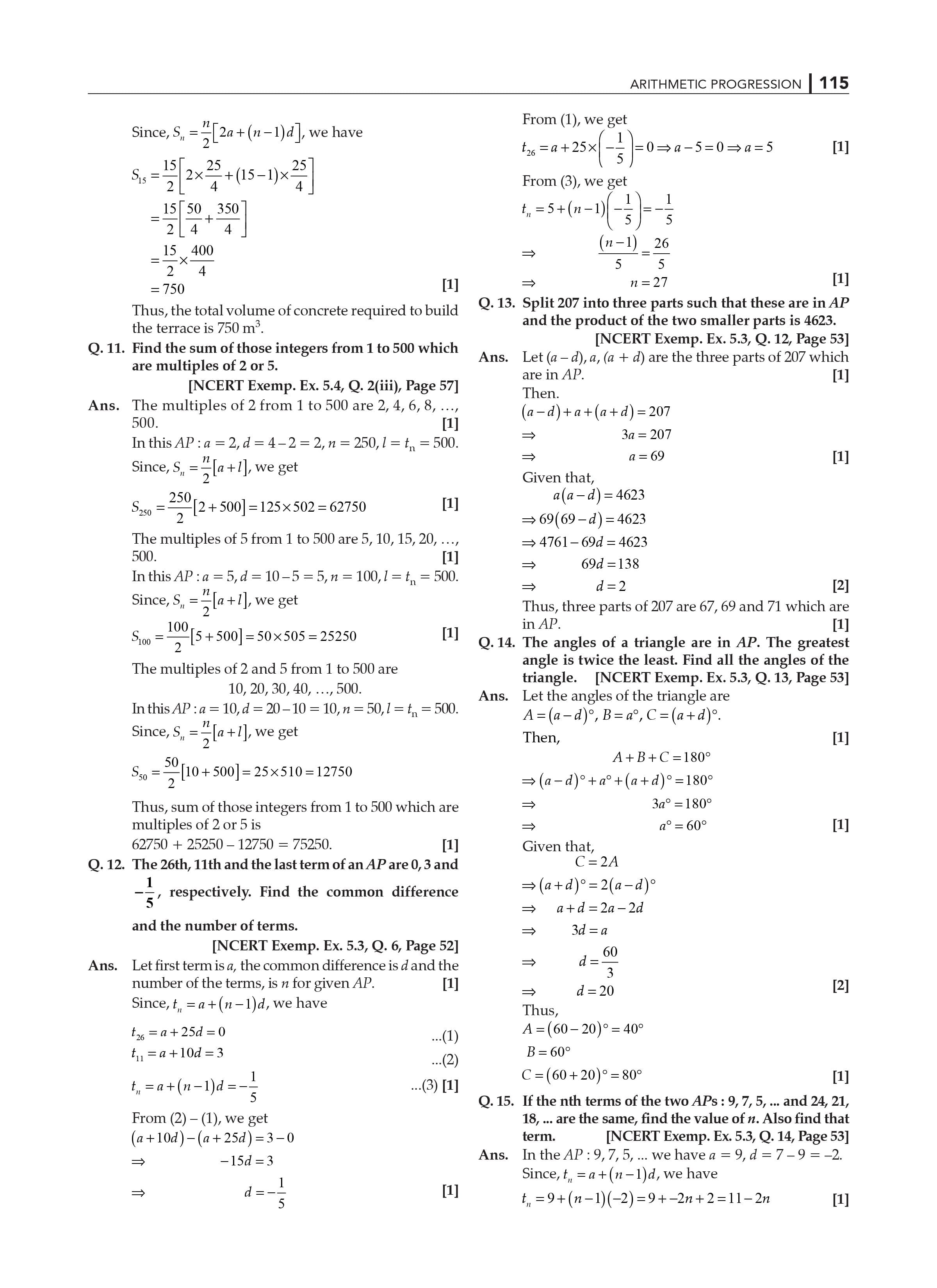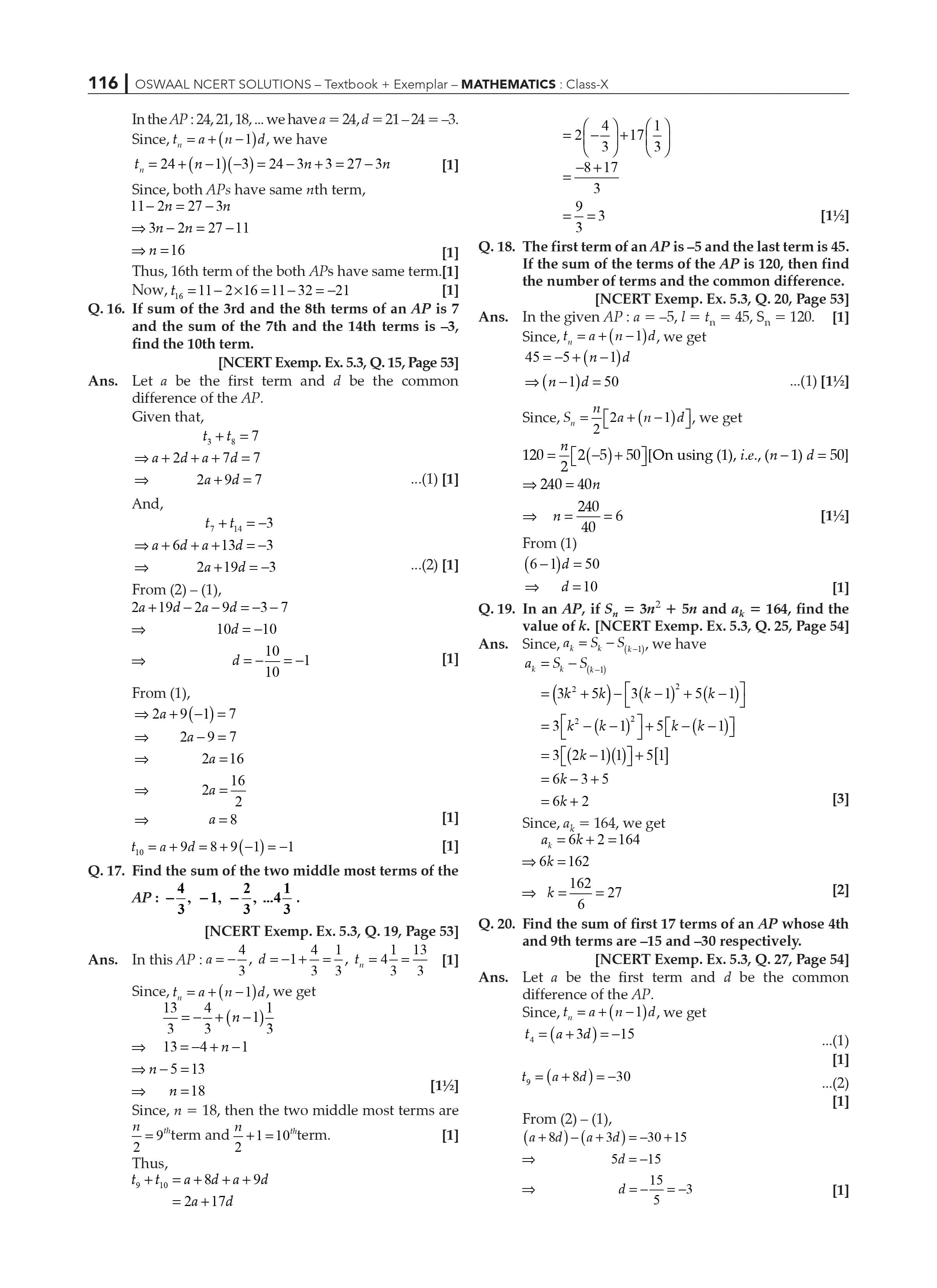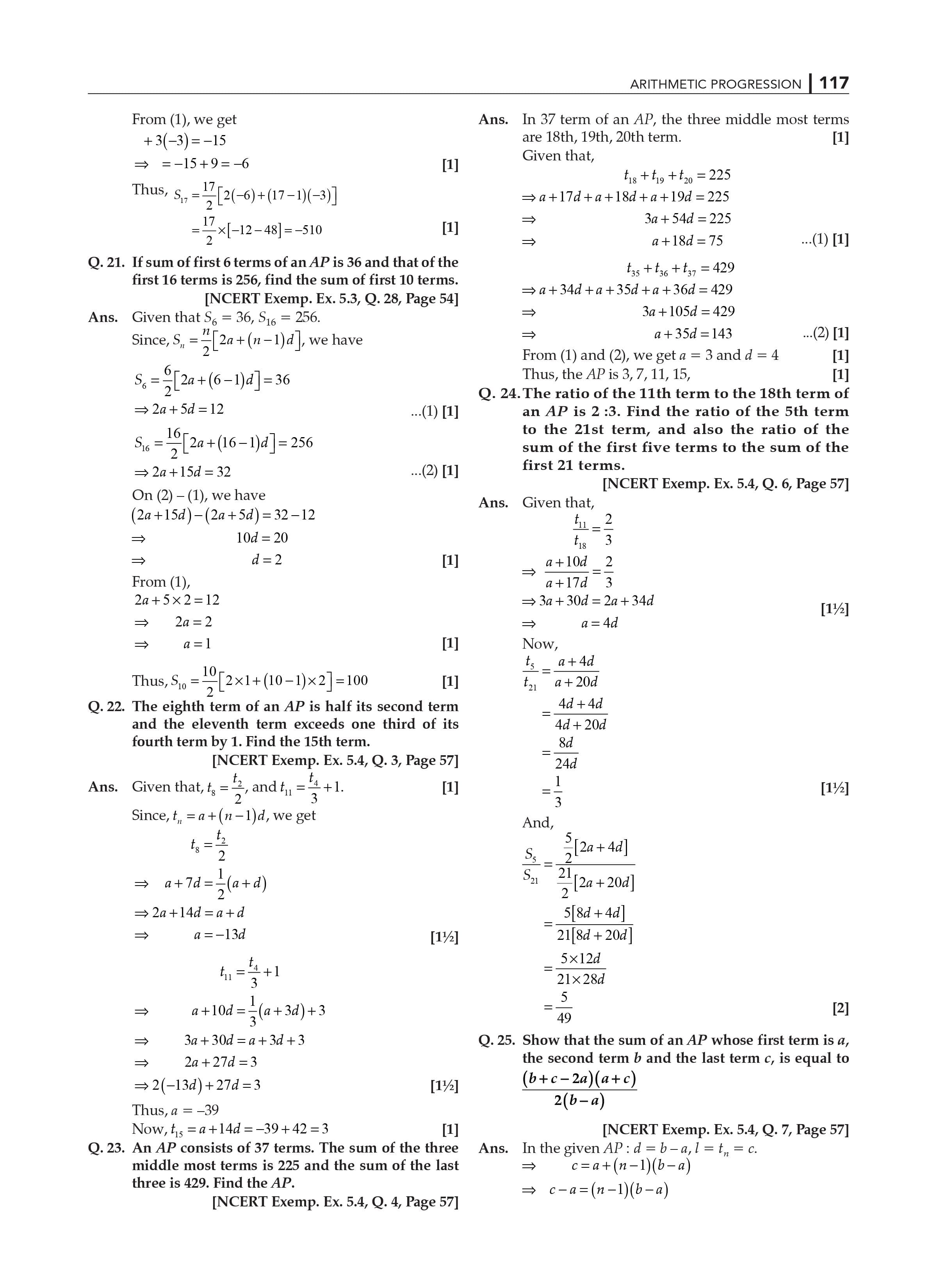NCERT Solutions for Class 10 Maths Exercise 5.1 Chapter 5.

Geometric Progression vs. Arithmetic Progression

In our website, the exercise solutions presented here are the product of extensive research and topic experts' experience. The answers to all of the questions in the NCERT Class 10 Maths textbook may be found on this NCERT Solution page. By completing this task, you will gain a better understanding of the introduction and fundamental principles involved in Arithmetic progression.

NCERT class 10 Maths textbook will be presented on this NCERT answer page. You will be able to answer simple questions about arithmetic progressions after studying this solution. Other Class 10 Maths Chapter 5- Arithmetic Progression exercise solutions can be found here.

20 questions in exercise 5.2 (1 fill in the blanks, 2 MCQs, 7 Short answer questions and 10 Long answer questions).

20 Questions (Exercise 5.3) (3 fill in the blanks, 4 daily life examples, and 13 descriptive type questions).

5 Questions (Exercise 5.4) (5 Long answer questions).

Introduction to the topics addressed in Exercise 5.1

The fundamentals of arithmetic progression

What does it do for you?

Answers the questions in Exercise 5.1 and aids in the learning of basic arithmetic principles.

You will be able to solve fewer complex arithmetic progression questions after studying this exercise page.

Allow you to get some practice answering questions.

You'll be able to answer a wide range of questions with varying degrees of difficulty.

Various examples from everyday life can assist you in properly comprehending the problem.

Continue to come to our website for help with CBSE Class 10 2023 exams. Students can find sample papers, question papers, notes, textbooks, films, animations, and practical preparation tips at this website.

For more information on the study resources we offer, visit the website for a tailored learning experience.

Subject matter experts developed model questions that covered all of the textbook's exercise questions to provide these solutions. They also focus on interpreting arithmetic solutions in an easy-to-understand manner for children.

Is it possible to answer all of the questions in the CBSE 2023 exams with the NCERT Solutions for Class 10 Maths Chapter 5?

Yes, answering all of the problems in the CBSE 2023 exams using the NCERT Solutions for Class 10 Maths Chapter 5 is adequate. They will be able to comprehend the fundamentals with easy if they practice this chapter. The guidelines were followed when creating these questions.

As a result, the students perform well on the CBSE 2023 examination.

Mathematical Progressions in Arithmetic Chapter 5: Key Features

You will become more familiar with important formulas by working through the NCERT Solutions.

As a starting point for answering questions about mathematical progression in competitive exams.

Has answers to all of the practice questions in the NCERT textbook.

Allow you to get some practice answering questions.

You'll be able to answer a wide range of questions with varying degrees of difficulty.

Allow you to get some practice answering questions.

You'll be able to answer a wide range of questions with varying degrees of difficulty.

Various examples from everyday life can assist you in properly comprehending the problem.

Continue to come to our website for help with CBSE Class 10 2023 exams.

NCERT Solutions for Class 10 Maths Chapter 5- Arithmetic Progression Exercise 5.2

The solutions are provided here to help you prepare for your Class 10 test. This website contains all of the answers to the questions in NCERT Class 10 Maths textbook Exercise 5.2.

NCERT Solutions for Class 10 Maths Chapter 5- Arithmetic Progression Exercise 5.2 will teach you how to solve arithmetic progression nth term questions. NCERT solutions will assist you in achieving perfection in all of the textbook's approaches.

Exercise 5.2 nth term of mathematical progression covers the following topics.

Examples that have been solved

Alternative approaches

What does it do for you?

• Make you practice a variety of question types.
• It teaches you how to solve arithmetic progression using various techniques.
• Improves your ability to solve arithmetic progression issues.
• It is the most effective study material with which to begin your exam preparation.
• Other class 10 Maths Chapter 5- Arithmetic Progression exercise solutions may be found here.

4 questions in exercise 5.1 (1 MCQ and 3 descriptive type questions)

20 questions in exercise 5.2 1 fill-in-the-blanks, 2 multiple-choice questions, 7 short answer questions, and 10 long answers.

The following is an overview of the topics covered in Exercise 5.2

Arithmetic progression's fundamentals

So, what exactly does it do for you?

Answers Exercise 5.2s questions and aids in the understanding of basic arithmetic principles.

After studying this exercise page, you will be able to solve fewer complex arithmetic progression questions.

Allow yourself the opportunity to practice answering questions.

You'll be able to respond to a variety of questions of varied difficulty.

Various examples from everyday life can help you understand the situation better.

Come back to our website for help with the first and second semester CBSE exams. This website provides students with sample papers, question papers, notes, textbooks, films, animations, and practical preparation recommendations.

NCERT Class 10 Maths Chapter 5 – Arithmetic Progression Exercise 5.2 Answers

Is it possible to use the NCERT Solutions for Class 10 Maths Chapter 5 to answer all of the questions in the CBSE 2023 exams?

Yes, utilizing the NCERT Solutions for Class 10 Maths Chapter 5 to answer all of the problems in the CBSE 2023 exams is sufficient. If students practice this chapter, they will be able to grasp the principles quickly. When designing these questions, the rules were followed.

As a result, they do well on the CBSE 2023 final test.

Key Features of Mathematical Progressions in Arithmetic Chapter 5 EXERCISE 5.2

• Answers to multiple-choice questions (MCQs) and long-answer questions on a variety of topics.
• Working through the NCERT Solutions will help you become more familiar with crucial formulas.
• As a place to start when answering questions question about the evolution of mathematics in competitive tests.
• All of the practice questions in the NCERT textbook have been answered.
• Allow yourself the opportunity to practice answering questions.
• You'll be able to respond to a variety of questions of varied difficulty.
• Allow yourself the opportunity to practice answering questions.
• You'll be able to respond to a variety of questions of varied difficulty.
• Various examples from everyday life can help you understand the situation better.

NCERT Solutions for Class 10 Maths Chapter 5- Arithmetic Progression Exercise 5.2

The solutions are provided here to help you prepare for your Class 10 test. This website contains all of the answers to the questions in NCERT Class 10 Maths textbook Exercise 5.2.

NCERT Solutions for Class 10 Maths Chapter 5- Arithmetic Progression Exercise 5.2 will teach you how to solve arithmetic progression nth term questions. NCERT solutions will assist you in achieving perfection in all of the textbook's approaches.

Exercise 5.2 nth term of mathematical progression covers the following topics.

Examples that have been solved

Alternative approaches

What does it do for you?

• Make you practice a variety of question types.
• It teaches you how to solve arithmetic progression using various techniques.
• Improves your ability to solve arithmetic progression issues.
• It is the most effective study material with which to begin your exam preparation.
• Other class 10 Maths Chapter 5- Arithmetic Progression exercise solutions may be found here.

20 questions in exercise 5.2 1 fill-in-the-blanks, 2 multiple-choice questions, 7 short answer questions, and 10 long answers.

The following is an overview of the topics covered in Exercise 5.2

Arithmetic progression's fundamentals

So, what exactly does it do for you?

Answers Exercise 5.2s questions and aids in the understanding of basic arithmetic principles.

After studying this exercise page, you will be able to solve fewer complex arithmetic progression questions.

Allow yourself the opportunity to practice answering questions.

You'll be able to respond to a variety of questions of varied difficulty.

Various examples from everyday life can help you understand the situation better.

Come back to our website for help with the first and second semester CBSE exams. This website provides students with sample papers, question papers, notes, textbooks, films, animations, and practical preparation recommendations.

NCERT Class 10 Maths Chapter 5 – Arithmetic Progression Exercise 5.2 Answer:

Is it possible to use the NCERT Solutions for Class 10 Maths Chapter 5 to answer all of the questions in the CBSE 2023 exams?

Yes, utilizing the NCERT Solutions for Class 10 Maths Chapter 5 to answer all of the problems in the CBSE Term II exams is sufficient. If students practice this chapter, they will be able to grasp the principles quickly. When designing these questions, the rules were followed.

As a result, they do well on the CBSE second-term final test.

Key Features of Mathematical Progressions in Arithmetic Chapter 5 EXERCISE 5.2

• Answers to multiple-choice questions (MCQs) and long-answer questions on a variety of topics.
• Working through the NCERT Solutions will help you become more familiar with crucial formulas.
• As a place to start when answering questions question about the evolution of mathematics in competitive tests.
• All of the practice questions in the NCERT textbook have been answered.
• Allow yourself the opportunity to practice answering questions.
• You'll be able to respond to a variety of questions of varied difficulty.
• Allow yourself the opportunity to practice answering questions.
• You'll be able to respond to a variety of questions of varied difficulty.
• Various examples from everyday life can help you understand the situation better.

Ex 5.3 NCERT Solutions for Class 10 Maths Chapter 5: Arithmetic Progression NCERT

A Solutions for Class 10 Maths the comprehensive answers to the difficulties are included in Exercise 5.3. These solutions are intended to aid students in their preparation for the CBSE Class 10 2023 examination.

Students should study this NCERT solution extensively in order to solve various types of questions of arithmetic progression. You will be better prepared to understand all types of questions that may be asked in class 10 2023 examinations if you complete these practice questions.

What is the benefit of it?

• It will assist you in understanding how to solve sums.
• It forces you to memories key formulas and approaches.
• Assists you with appropriately scoring all of the questions on arithmetic progression.
• NCERT Solutions for Class 10 Maths Chapter 5- Arithmetic Progression Exercise 5.3 are available in PDF format.

The subjects addressed in Exercise 5.3 Arithmetic progression's principles are summarized below.

So, what does it actually do for you?

• Answers the questions in Exercise 5.3 and aids in the comprehension of basic arithmetic ideas.
• You will be able to solve fewer complex arithmetic progression questions after studying this exercise page.
• Allow yourself to get some practice with responding questions.
• You'll be able to react to a wide range of difficult queries.
• Various examples from ordinary life can aid in your comprehension of the problem.
• Return to the website for assistance with the CBSE first and second semester exams. Sample papers, question papers, notes, textbooks, films, animations, and practical preparation tips are all available on this website for students.

Arithmetic's Key Characteristics of Mathematical Progressions

• On a variety of themes, answers to multiple-choice questions (MCQs) and long-answer questions.
• Working through the NCERT Solutions can help you gain a better understanding of important formulas.
• When answering inquiries concerning the evolution of mathematics in competitive assessments, this is a good place to start.
• The NCERT textbook's practice questions have all been solved.
• Allow yourself to get some practice with responding questions.
• You'll be able to react to a wide range of difficult queries.
• Allow yourself to get some practice with responding questions.
• You'll be able to react to a wide range of difficult queries.
• Various examples from ordinary life can aid in your comprehension of the problem.

NCERT Solutions for Class 10 Maths Exercise 5.4 Chapter 5.

NCERT Solutions for Class 10 Maths Chapter 5: Arithmetic Progression Exercise 5.4 is published here to assist students in tackling the long answer questions found in NCERT Class 10 Maths textbook exercise 5.4. Students must become familiar with this exercise solution in order to solve long answer problems.

Long answers to mathematical problems are frequently missed by students. Students should read this exercise solution thoroughly in order to understand how to tackle long answer issues. You would be able to eliminate your worries in solving long answer questions by studying NCERT Solutions.

Exercise 5.4 Sum of First n Terms of an AP Solved covers the following topics.

What does it do for you?

• Assist you in figuring out how to solve long-answer questions.
• Studying this answer will assist you in getting good grades on the exam.
• It forces you to memories several formulas and methods for solving arithmetic progression issues.
• NCERT Solutions for Class 10 Maths.

What is the advantage of doing so?

• It will assist you in learning how to solve math problems.
• It compels you to remember crucial formulas and strategies.
• Assists you in correctly scoring all of the arithmetic progression questions.

NCERT Solutions for Class 10 Maths Chapter 5: Key Features Progressions in arithmetic

• Answers to a variety of topics, including MCQs and long-answer questions.
• By working through the NCERT Solutions, you will get more familiar with important formulas.
• As a starting point for solving arithmetic progression questions in competitive exams.
• Has answers to all of the NCERT textbook's practice questions.
• Give you the opportunity to practice answering questions.
• You can solve a variety of questions of varied degrees of difficulty.
• Various examples

Give you the opportunity to practice answering questions

You can solve a variety of questions of varied degrees of difficulty.

Different examples from everyday life will help you fully comprehend the issue.

Continue to visit our website for comprehensive help with CBSE Class 10 board exams. Students can access a variety of sample papers, question papers, notes, textbooks, videos, animations, and practical preparation suggestions to help them to do well in the CBSE class 10 exams in 2022-2023.

Students can also study the CBSE syllabus's other solutions to understand the types of questions that will be asked.

An organized approach to grasping topics and solving problems is required to pass the Class 10 Mathematics tests. The RD Sharma Solutions meets these requirements, allowing a student to achieve high results in the qualification exam. Furthermore, our expert team at Oswaal Books has produced answers in simple language with adequate explanations to provide understanding on the issues in the chapter.

The chapter Arithmetic Progressions in the Solutions Class 10 is a fun chapter where students may easily get good grades. However, due to several misconceptions, not all pupils will be able to obtain those grades. The RD Sharma Solutions for Class 10 provide descriptive answers to all six tasks in order to assist students. The following are the main points of this chapter:

• Sequences should be understood.
• Progressions in Arithmetic
• Writing the algebraic formula for the sequence's terms to describe it
• In an P., find the sum of terms.
• Various word problems relating to Arithmetic Progressions must be solved.

 Extra Information: NCERT Exemplar for Class 10 Maths Chapter 5 CBSE Notes for Class 10 Maths Chapter 5

Frequently Asked Questions: NCERT Solutions Class 10 Maths Chapter 5 Arithmetic Progression

Is it vital to study NCERT Solutions for Class 10 Maths Chapter 5 for the exam?

Yes, NCERT Solutions for Class 10 Maths Chapter 5 are essential for improving problem-solving skills in preparation for exams. This chapter delves into Arithmetic Progression in depth, which is vital for both board exams and higher education. As a result, learning these concepts is easier for kids, and their doubts are swiftly cleared. This chapter includes key questions that may appear on board exams.

Give a brief overview of NCERT Class 10 Maths Chapter 5 Solutions?

There are six exercises in NCERT Solutions for Class 10 Maths Chapter 5. The subject of this chapter is

covers a variety of subjects relating to the Arithmetic Progression, including:

1. Recognizing sequences
2. Arithmetic Progressions are a type of arithmetic progression.
3. Writing the algebraic formula for the sequence's terms to describe it
4. In an A.P., find the sum of terms.
5. Solving a variety of word problems involving Arithmetic Progressions.

What are the most significant advantages of studying NCERT Solutions for Class 10 Maths Chapter 5?

NCERT Solutions for Class 10 Maths Chapter 5answers provide in-depth understanding of concepts for effective exam preparation. This makes it simple to dispel any doubts about Arithmetic Progression. By practicing these answers on a regular basis, students may quickly solve any sort of question.

NCERT Solutions for Class 10 Maths Chapter 5: Frequently Asked Questions?

• Maths Chapter 5 must be learned.
• Maths Chapter 5 can help you get good grades in your Class 10 second-term exams. These solutions were created by subject matter specialists of our team who compiled model questions that covered all of the textbook's exercise problem with explanation in detailed. They also concentrate on deciphering Math solutions in a style that is simple for kids to comprehend.

Is it possible to use the NCERT Solutions for Class 10 Maths Chapter 5 to answer all of the questions in the CBSE board exams?

Yes, our experts solve the all problems of the NCERT Solutions for Class 10 Maths Chapter 5 to answer all of the problems in the CBSE exams is sufficient. By rehearsing the chapter, they will be able to grasp the principles with ease. These questions were created in accordance with the guidelines.

As a result, students’ work hard and give a better result in the CBSE final examination.